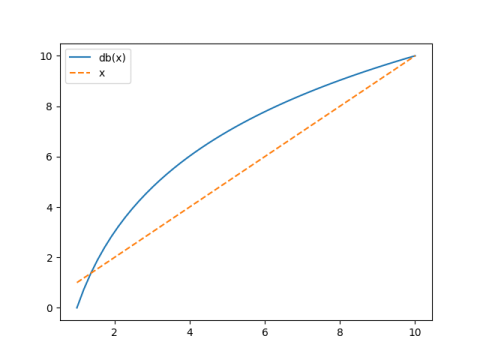Logarithms in all bases are proportional. Specifically

loga(x) = logb(x) / logb(a)

for any bases a and b. One way to read this is to say that if you already know about logarithms base b, logarithms base a aren’t anything fundamentally new . They’re proportional to logarithms base b, and in fact the proportionality constant is 1/logb(a).

This post would like to turn the discussion above around: a multiple of a logarithm is just a logarithm in another base, and it’s easy to find what that new base is.

The most famous function that is a multiple of a logarithm might be the function that converts numbers to decibel scale:

f(x) = 10 log10(x).

This is a multiple of a logarithm, and so there is some base b such that f(x) is log base b.

It turns out b is 101/10. That is, decibel levels are logarithms base

b = 101/10 = 1.2589

So multiplying something by 1.2589 increases its decibel level by 1. That is, making something 1/4 larger increases its decibel level by slightly less than 1. 

Where did this b come from? How can we show that it’s correct?

Let’s start by finding the inverse of f. The function f does two things to x: it takes the log base 10, then multiplies by 10. Its inverse undoes things in the opposite order, i.e. it divides by 10 and raises 10 to the result.

f -1(x) = 10x/10.

Now

10x/10 = (101/10)x

and so the inverse of f is the function that takes 101/10 to the power x. That means f must be the logarithm of x in base 101/10.

For possible future reference, let’s solve this problem in a little more generality, converting any multiple of a logarithm in any base to logarithm in another base.

If

f(x) = c loga(x) = logb(x)

then

f -1(x) = ax/c = (a1/c)x = bx

and so

b = a1/c.

## Related posts

 It is convenient to use logarithms in different bases in different contexts even though they’re all proportional, just as it’s convenient to measure angles sometimes in degrees and sometimes in radians, even thought degrees and radians are proportional.

 So why think of decibels as 10 log10 rather that log base 101/10? Because the former is much easier to conceptualize. Natural logarithms are easier to work with theoretically, but logarithms base 10 are easier to work with mentally.

And why multiply the decimal log by 10? Because then for single digit numbers, the original value and its decibel value have the same order of magnitude. And when x = 10, the two are equal.